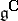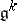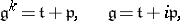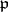# Cartan decomposition

(diff) ← Older revision | Latest revision (diff) | Newer revision → (diff)
Jump to: navigation, search

A representation of a real non-compact semi-simple Lie algebra(cf. Lie algebra, semi-simple) as a direct sum of vector spaces (*). Ifdenotes the complexification (complex envelope) of(cf. Complexification of a Lie algebra), then there exists ina real compact subalgebraof the same dimension assuch that the following decompositions into direct sums of vector spaces hold:(*)

whereis the subalgebra of invariant elements of some involutory automorphism (involution)ofandis the set of anti-invariant elements of. The second formula is the Cartan decomposition of(see ). The Cartan decomposition reduces the classification of real non-compact semi-simple Lie algebras to that of compact semi-simple Lie algebras and involutory automorphisms in them.

How to Cite This Entry:
Cartan decomposition. Encyclopedia of Mathematics. URL: http://encyclopediaofmath.org/index.php?title=Cartan_decomposition&oldid=17328
This article was adapted from an original article by A.S. Fedenko (originator), which appeared in Encyclopedia of Mathematics - ISBN 1402006098. See original article